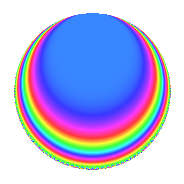Properties

 Label 3600.1.bh.aLevel 3600 Weight 1 Character orbit 3600.bh Analytic conductor 1.797 Analytic rank 0 Dimension 2 Projective image $$D_{2}$$ CM/RM disc. -3, -15, 5 Inner twists 8

Related objects

Newspace parameters

 Level: $$N$$ = $$3600 = 2^{4} \cdot 3^{2} \cdot 5^{2}$$ Weight: $$k$$ = $$1$$ Character orbit: $$[\chi]$$ = 3600.bh (of order $$4$$ and degree $$2$$)

Newform invariants

 Self dual: No Analytic conductor: $$1.79663404548$$ Analytic rank: $$0$$ Dimension: $$2$$ Coefficient field: $$\Q(i)$$ Coefficient ring: $$\Z[a_1, \ldots, a_{49}]$$ Coefficient ring index: $$1$$ Projective image $$D_{2}$$ Projective field Galois closure of $$\Q(\sqrt{-3}, \sqrt{5})$$ Artin image size $$16$$ Artin image $OD_{16}$ Artin field Galois closure of 8.4.14580000000.1

$q$-expansion

The $$q$$-expansion and trace form are shown below.

 $$f(q)$$ $$=$$ $$q$$ $$+O(q^{10})$$ $$q$$ $$-2 i q^{19}$$ $$+ 2 q^{31}$$ $$-i q^{49}$$ $$+ 2 q^{61}$$ $$+ 2 i q^{79}$$ $$+O(q^{100})$$ $$\operatorname{Tr}(f)(q)$$ $$=$$ $$2q$$ $$\mathstrut +\mathstrut O(q^{10})$$ $$2q$$ $$\mathstrut +\mathstrut 4q^{31}$$ $$\mathstrut +\mathstrut 4q^{61}$$ $$\mathstrut +\mathstrut O(q^{100})$$

Character Values

We give the values of $$\chi$$ on generators for $$\left(\mathbb{Z}/3600\mathbb{Z}\right)^\times$$.

 $$n$$ $$577$$ $$901$$ $$2801$$ $$3151$$ $$\chi(n)$$ $$i$$ $$1$$ $$1$$ $$1$$

Embeddings

For each embedding $$\iota_m$$ of the coefficient field, the values $$\iota_m(a_n)$$ are shown below.

For more information on an embedded modular form you can click on its label.

Label $$\iota_m(\nu)$$ $$a_{2}$$ $$a_{3}$$ $$a_{4}$$ $$a_{5}$$ $$a_{6}$$ $$a_{7}$$ $$a_{8}$$ $$a_{9}$$ $$a_{10}$$
2593.1
 − 1.00000i 1.00000i
0 0 0 0 0 0 0 0 0
3457.1 0 0 0 0 0 0 0 0 0
 $$n$$: e.g. 2-40 or 990-1000 Significant digits: Format: Complex embeddings Normalized embeddings Satake parameters Satake angles

Inner twists

Char. orbit Parity Mult. Self Twist Proved
1.a Even 1 trivial yes
3.b Odd 1 CM by $$\Q(\sqrt{-3})$$ yes
5.b Even 1 RM by $$\Q(\sqrt{5})$$ yes
15.d Odd 1 CM by $$\Q(\sqrt{-15})$$ yes
5.c Odd 2 yes
15.e Even 2 yes

Hecke kernels

This newform can be constructed as the kernel of the linear operator $$T_{7}$$ acting on $$S_{1}^{\mathrm{new}}(3600, [\chi])$$.